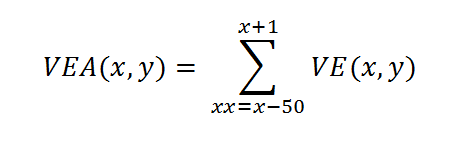## Plate Finding

There are several published methods for locating a number plate in an image. Some methods attempt to find blocks or rectangles in the image, or plate edges. These can be unreliable because in many cases the plate is light in color and is attached to a light colored area which obscures the edges.

My method relies on identifying the key characteristic of a number plate – the concentration of characters with strong light-on-dark edges. My algorithm has been developed to locate strong edges which are spaced proportionally given the expected size of the plate relative to the overall image. A strong edge is defined as a vertical line where adjacent pixels have high luminance deltas, relative to other areas of the scene.

The first step is to convert the color (RGB) image into a luminance array, were the definition of luminance is the standard conversion from RGB to YUV, but the Y component is scaled up to whole number integers (multiplied by 1000). An intensity-colorized version of the luminance array is show below (where intensity-colorization is used to express luminance values that range from 0 to 1000).

In order to make the processing as efficient as possible, the second step in the processing chain is to sub-scale the image down as small as possible without loosing too much critical information. The basis for determining the scaling factors is setting assumptions on the ratio of plate height (and character height) to image height (or widths). A sample sub-scaled luminance array is shown below (again with intensity-colorization).

Once the image is sub-scaled, then the edge processing algorithm is applied.

In this method, we will find the plate by locating the highest concentration of edge energy, where our definition of edge-energy are edges that meet a luminance difference threshold, and a spacial concentration threshold.  Here is math:

Define E(x,y) as a two dimensional array which we will define as the concentrated-edge-energy-map.Define the VEA as vertical energy in an area around the point (x,y).  Where VE(x,y) is defined as the vertical edge energy at on a vertical line 10 pixels tall at given column x:And VEA(x,y) is calculated asAnd the resulting area-concentrated-edge-energy map is show below (intensity-colorized):

As you can see, the location of the plate is stands out dramatically from the surroundings. Now the only thing left to do is find the brightest spot, then do additional analysis in the full resolution luminance array to isolate the plate edges.

This algorithm works very well at finding multiple plates in an image as well: